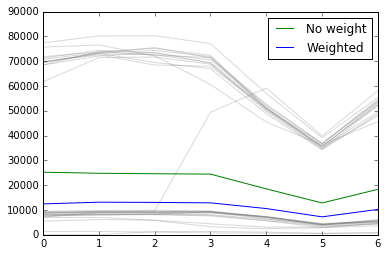In :
%matplotlib inline

import aylien_news_api
from aylien_news_api.rest import ApiException
import pandas as pd
import matplotlib.pyplot as plt
import sys
import datetime
import numpy as np


### Global script configs¶

In :
# start and end of period
start = "NOW-300DAY"
end = "NOW"
# summarize key. possible values = day, week, month
summarize = "week"
# count of parts to split each bucket (for weighted average)
weight_steps = 4
# AYLIEN News API keys
aylien_news_api.configuration.api_key['X-AYLIEN-NewsAPI-Application-ID'] = ''
aylien_news_api.configuration.api_key['X-AYLIEN-NewsAPI-Application-Key'] = ''


### Main() { ... }¶

In :
if (summarize == "month"):
period = "+1DAY"
chunks = 30
elif (summarize == "week"):
period = "+1DAY"
chunks = 7
elif (summarize == "day"):
period = "+1HOUR"
chunks = 24
else:
print('Invalid summarize value')
sys.exit(0)

api_instance = aylien_news_api.DefaultApi()

try:
print('fetching data...')

# List time series
api_response = api_instance.list_time_series(published_at_start = start,
published_at_end = end,
period = period)

data = api_response.time_series
chunk =  * chunks
tmp_chunk =  * chunks
history_tmp_chunks = []
i = 0
i_total = 0
skip = True

for d in data:
# EST timezone
d.published_at = d.published_at - datetime.timedelta(hours=+5)

# we should start adding values when we are on the first member of our series
# e.g. if the summarize is weekly, we should start adding values on Monday
# e.g. if the summzrize is daily, we should start adding values when it's 00:00
if (summarize == "week" and d.published_at.strftime("%A") == 'Monday'):
skip = False
elif (summarize == "day" and
d.published_at.hour == 0):
skip = False
elif (summarize == "month"  and d.published_at.day == 1):
skip = False

# also, we should skip adding values if don't have enough members left to add (based on chunk size)
if (data.index(d) + chunks > len(data)):
skip = True

if skip:
print('skipping...', d.published_at.strftime('%A'), 'at ' + str(d.published_at.hour))
continue

if (i == chunks):
plt.plot(tmp_chunk, color='gray', alpha=0.3)
i = 0
i_total += 1
history_tmp_chunks.append(tmp_chunk[:])

chunk[i] += d.count
tmp_chunk[i] = d.count

i += 1

weighted_col = []
# calculating the weight
for w in range(chunks):
col = np.array(history_tmp_chunks)[:, w]
col_min, col_max = min(col), max(col)
step_val = (col_max - col_min) / weight_steps

# because the col_min is only the first element of steps
steps = [col_min]

# the score of each step
steps_score =  * weight_steps

# preparing the steps array
for step in range(weight_steps):
steps.append(steps[-1] + step_val)

for i in range(len(col)):
for j in range(len(steps)):

if j == 0:
continue

if steps[j - 1] <= col[i] < steps[j]:
steps_score[j - 1] += 1

weighted_col_tmp = []
weighted_col_sum = []
for i in range(len(col)):
for j in range(len(steps)):

if steps[j - 1] <= col[i] < steps[j]:
weighted_col_tmp.append(col[i] * steps_score[j - 1])
weighted_col_sum.append(steps_score[j - 1])

weighted_col.append(np.sum(weighted_col_tmp) / np.sum(weighted_col_sum))

print('score array:', steps_score)

weighted, = plt.plot(weighted_col, label='Weighted')

j = 0
for c in chunk:
if (chunk[j] != 0):
chunk[j] = c / i_total

j += 1

no_weight, = plt.plot(chunk, label='No weight')

plt.legend(handles=[no_weight, weighted])

except ApiException as e:
print("Exception when calling DefaultApi->list_time_series: %s\n" % e)

fetching data...
skipping... Tuesday at 9
skipping... Wednesday at 9
skipping... Thursday at 9
skipping... Friday at 9
skipping... Saturday at 9
skipping... Sunday at 9
skipping... Tuesday at 9
skipping... Wednesday at 9
skipping... Thursday at 9
skipping... Friday at 9
skipping... Saturday at 9
skipping... Sunday at 9
score array: [29, 0, 0, 11]
score array: [29, 0, 0, 11]
score array: [29, 0, 0, 11]
score array: [28, 0, 1, 11]
score array: [28, 0, 0, 12]
score array: [28, 0, 0, 12]
score array: [28, 0, 0, 12]